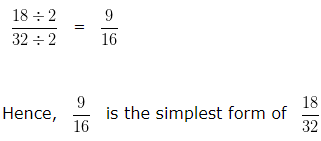# Fraction in Simplest Form

Fraction in Simplest Form ( Simplify Fractions ) – A Fraction is said to be in simplest form when the numerator and denominator of a fraction has only common factor i.e, 1

In other words, To simplify a fraction in simplest form we divide the numerator and denominator of a fraction by their H.C.F ( Highest Common Factor ).

## Fraction in Simplest Form Examples

Example 1

Convert  15/45  into its Simplest Form?

Explanation :

Finding HCF of 15 and 45.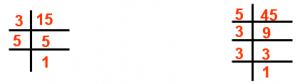Factors of 15 are  35

Factors of 45 are  53,  3

HCF of 15 and 45 = 3 x 5 = 15

Divide Numerator and Denominator by their HCF i.e, 15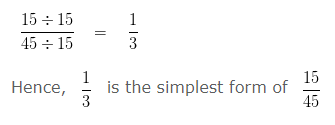Example 2

Convert 70/30 into its simplest form.

Explanation:

Given Fraction:
70/30

In order to convert a fraction, into its simplest form, we first find the HCF of the Numerator and Denominator
HCF of 70 and 30 is 10

Thereafter, we divide both the Numerator and Denominator by their HCF i.e, 10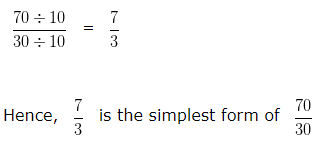Example 3

Convert 24/54 into its simplest form.

Explanation:

Given Fraction:
24/54

In order to convert a fraction, into its simplest form, we first find the HCF of the Numerator and Denominator
HCF of 24 and 54 is 6

Thereafter, we divide both the Numerator and Denominator by their HCF i.e, 6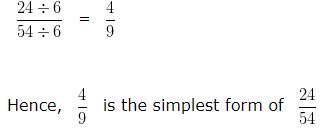Example 4

Convert 16/12 into its simplest form.

Explanation:

Given Fraction:
16/12

In order to convert a fraction, into its simplest form, we first find the HCF of the Numerator and Denominator
HCF of 16 and 12 is 4

Thereafter, we divide both the Numerator and Denominator by their HCF i.e, 4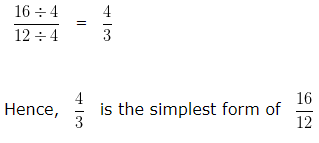Example 5

Convert 18/32 into its simplest form.

Explanation:

Given Fraction:
18/32

In order to convert a fraction, into its simplest form, we first find the HCF of the Numerator and Denominator
HCF of 18 and 32 is 2

Thereafter, we divide both the Numerator and Denominator by their HCF i.e, 2